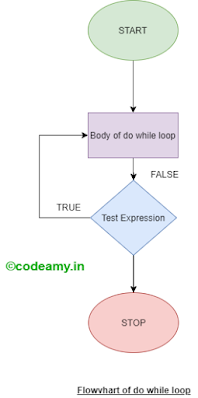# do while - C Program, Syntax and Flowchart

In this tutorial, You learn about the do-while loop in c programming then understand flowchart and c program. In the previous tutorial learned while loop & for loop in this both the test condition occurs at the top but in this test expression occurs at the bottom.

Loop is used to repeat the block of statements until the given test expression or test condition return false. Also, learn about c flow controls.

IMPORTANT NOTE: In this, condition occurs at the end of the loop, so the body (All Statements) in the loop executes once before the condition is tested.

## do while Loop Syntax

Here I shared do while loop syntax and you easily understand how loop executes and statements loop inside the loop body.

``````variable initialization //eg. i=1;
do{
//All Statements
// Variable Increment/Decrement eg. i++;
}while( test expression )
``````

## do-while Flowchart Diagram

Below I shared the flowchart diagram for the do-while loop in c programming. So, It is easy for you to understand the flowchart.do while flowchart

## Program

``````#include<stdio.h>
int main() {
int count=0;
do {
printf("Value of count is: %d\n", count);
count++;
}while (count<3);
return 0;
}
``````

When the above code is compiled and executed, it produces the following output

``````
Value of count is:1
Value of count is:2
``````

In the Above article, We discuss do while in c programming and flowchart, and if you have any doubt in the article then comment below.

Happy Coding 😊

If you have any doubts, Please let me know